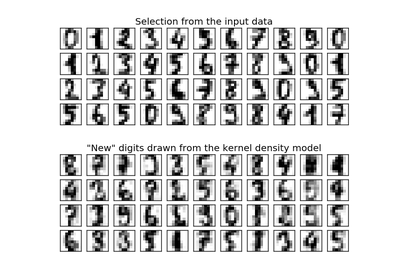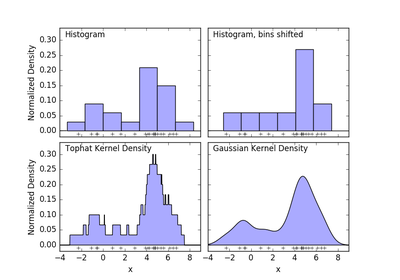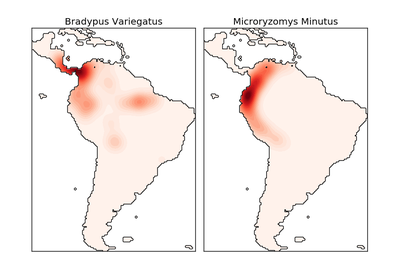# `sklearn.neighbors`.KernelDensity¶

class `sklearn.neighbors.``KernelDensity`(bandwidth=1.0, algorithm='auto', kernel='gaussian', metric='euclidean', atol=0, rtol=0, breadth_first=True, leaf_size=40, metric_params=None)[source]

Kernel Density Estimation

Read more in the User Guide.

Parameters: bandwidth : float The bandwidth of the kernel. algorithm : string The tree algorithm to use. Valid options are [‘kd_tree’|’ball_tree’|’auto’]. Default is ‘auto’. kernel : string The kernel to use. Valid kernels are [‘gaussian’|’tophat’|’epanechnikov’|’exponential’|’linear’|’cosine’] Default is ‘gaussian’. metric : string The distance metric to use. Note that not all metrics are valid with all algorithms. Refer to the documentation of `BallTree` and `KDTree` for a description of available algorithms. Note that the normalization of the density output is correct only for the Euclidean distance metric. Default is ‘euclidean’. atol : float The desired absolute tolerance of the result. A larger tolerance will generally lead to faster execution. Default is 0. rtol : float The desired relative tolerance of the result. A larger tolerance will generally lead to faster execution. Default is 1E-8. breadth_first : boolean If true (default), use a breadth-first approach to the problem. Otherwise use a depth-first approach. leaf_size : int Specify the leaf size of the underlying tree. See `BallTree` or `KDTree` for details. Default is 40. metric_params : dict Additional parameters to be passed to the tree for use with the metric. For more information, see the documentation of `BallTree` or `KDTree`.

Methods

 `fit`(X[, y]) Fit the Kernel Density model on the data. `get_params`([deep]) Get parameters for this estimator. `sample`([n_samples, random_state]) Generate random samples from the model. `score`(X[, y]) Compute the total log probability under the model. `score_samples`(X) Evaluate the density model on the data. `set_params`(\*\*params) Set the parameters of this estimator.
`__init__`(bandwidth=1.0, algorithm='auto', kernel='gaussian', metric='euclidean', atol=0, rtol=0, breadth_first=True, leaf_size=40, metric_params=None)[source]
`fit`(X, y=None)[source]

Fit the Kernel Density model on the data.

Parameters: X : array_like, shape (n_samples, n_features) List of n_features-dimensional data points. Each row corresponds to a single data point.
`get_params`(deep=True)[source]

Get parameters for this estimator.

Parameters: deep : boolean, optional If True, will return the parameters for this estimator and contained subobjects that are estimators. params : mapping of string to any Parameter names mapped to their values.
`sample`(n_samples=1, random_state=None)[source]

Generate random samples from the model.

Currently, this is implemented only for gaussian and tophat kernels.

Parameters: n_samples : int, optional Number of samples to generate. Defaults to 1. random_state : RandomState or an int seed (0 by default) A random number generator instance. X : array_like, shape (n_samples, n_features) List of samples.
`score`(X, y=None)[source]

Compute the total log probability under the model.

Parameters: X : array_like, shape (n_samples, n_features) List of n_features-dimensional data points. Each row corresponds to a single data point. logprob : float Total log-likelihood of the data in X.
`score_samples`(X)[source]

Evaluate the density model on the data.

Parameters: X : array_like, shape (n_samples, n_features) An array of points to query. Last dimension should match dimension of training data (n_features). density : ndarray, shape (n_samples,) The array of log(density) evaluations.
`set_params`(**params)[source]

Set the parameters of this estimator.

The method works on simple estimators as well as on nested objects (such as pipelines). The latter have parameters of the form `<component>__<parameter>` so that it’s possible to update each component of a nested object.

Returns: self :

## Examples using `sklearn.neighbors.KernelDensity`¶Kernel Density EstimationSimple 1D Kernel Density EstimationKernel Density Estimate of Species Distributions